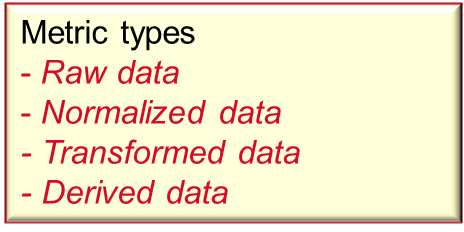# Performance measurement metrics

Metrics are the output from performance measurements.

To produce metrics with high quality all captured performance data must comply to a number of requirements.

This page explains different types performance data and the requirements on captured performance data.

## General requirements

Performance metrics must comply to five general requirements.General requirements on metrics

### 1. Uniquely identified

The naming of metrics must be unique to avoid name conflicts

### 2. Understandable

Metrics should be accompanied by specifications that make them easy to understand.

### 3. Comparable

Metrics should be accompanied by recording conditions and processing methods to be comparable.

### 4. Repeatable

Metrics should specify test case specifications, test tool specifications, and test object specifications.

This is a basic requirement for regression tests of performance.

### 5. Accurate

Metrics should be accompanied by data recording methods and configurations .

To top of page

## Metric identifiers

Every measurement value must have three identifying attributes.Metric identifiers

### 1. What

What does a metric value represent?

### 2. Where

Where is the metric value captured, i.e. where is the measurement point of a metric value?

### 3. When

When was the metric value captured? Performance measurements generate long time series of measurement data. Therefore is the point in time critical for comparisons with other metrics.

Performance metrics are of no value if they are not accompanied by the three identifiers, since you can't tell what they represent.

To top of page

## Metric types

Metrics belong to one of four types.Metric types

### 1. Raw data

Raw data are captured data in its original format. Raw data can in some cases be used as metrics in its final format, such as response time.

### 2. Normalized data

Normalized data are metric figures that relates to normalizing factor, such as transactions per second.

### 3. Transformed data

Transformed data are metric figures transformed from the recorded value format to another value format, such as memory size transformed to a percentage of total memory.

### 4. Derived data

Derived data are metric figures resulting from computations based on other captured metrics. Key Performance Indicators are typically derived metrics.

To top of page

## Metric formats

Metrics values are described with three format attributes.Metric formats

### 1. Value type

Value type tells what a metric value represents, such as time, counters, or other.

### 2. Unit

Is the quantity format a metric value, such as millisecond for time.

### 3. Accuracy

The precision of a metric value.

To top of page

## Measurement conditions

Valid measurement conditions are fundamental to the quality of metric values.Measurement conditions

The measured conditions on the SUT when requested measurement values were captured are used to separate valid measurement values from invalid.

To top of page

Proceed to Performance Measurement Conditions